# Difference between revisions of "Binder cumulant"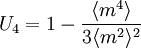$U_4 = 1- \frac{\langle m^4 \rangle }{3\langle m^2 \rangle^2 }$
In the thermodynamic limit, where the system size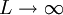$L \rightarrow \infty$,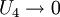$U_4 \rightarrow 0$ for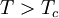$T > T_c$, and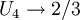$U_4 \rightarrow 2/3$ for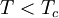$T < T_c$. Thus, the function is discontinuous in this limit --- the useful fact is that curves corresponding to different system sizes (which are, of course, continuous) all intersect at approximately the same temperature, which provides a convenient estimatation of the critical temperature.